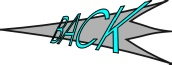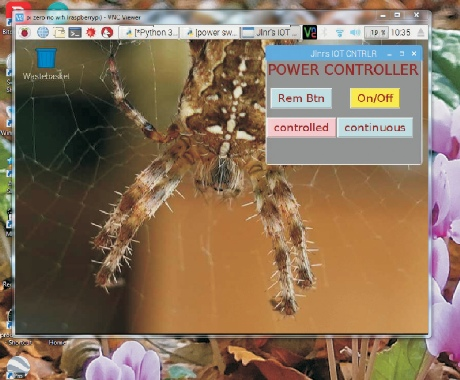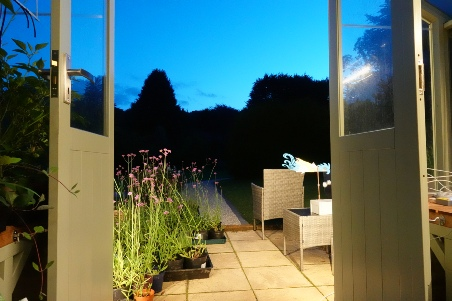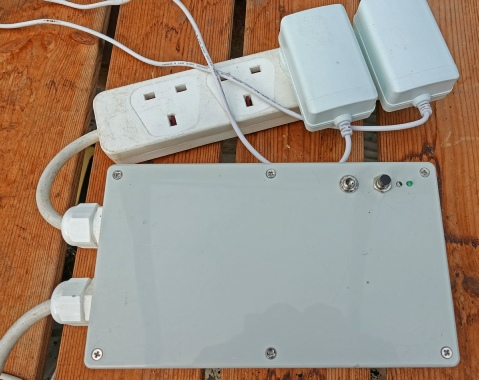﻿ Greenhouse lighting, remote switch software.Under Construction

Greenhouse lighting remote switch Python software

# GUI to control power switch

# by Julian Rogers 3.10.19

# "power switch 1a"

from tkinter import *   #GUI

import RPi.GPIO as GPIO

GPIO.setmode(GPIO.BCM)

GPIO.setwarnings(False)

GPIO.setup(5, GPIO.OUT)     #red LED, pilot light for mains on

GPIO.setup(19, GPIO.OUT)    #green LED, flashes if running

GPIO.setup(26, GPIO.OUT)    #controls mains relay

GPIO.output(5, False)

GPIO.output(19, False)

GPIO.output(26, False)

GPIO.setup(6, GPIO.IN)      #push to break button to manually turn on/off

GPIO.setup(16, GPIO.IN)     #detects override switch in "controlled position"

GPIO.setup(20, GPIO.IN)     #detects override switch in "continuous" position

root = Tk()     # create the root window

# modify the window

root.title("Jlnr's IOT CNTRLR")

root.geometry("300x200")

root.configure(bg = "gray")

# create a frame

app = Frame(root)

app.configure(bg = "gray")

app.grid()

title_lab = Label(app, text = "POWER CONTROLLER", font = ("Arial Bold", 20), fg = "maroon", bg = "gray")

title_lab.grid(row = 1, column = 1, columnspan = 8)

blank_lab = Label(app, bg = "gray")

blank_lab.grid(row = 2, column = 1)

sys_3_but = Button(app, text = "On/Off", font = ("Arial", 16), fg = "maroon", bg = "light blue")

sys_3_but.grid(row = 3, column = 2)

def on_off_3():

but_col = sys_3_but.config('bg')[-1]    #use button colour as a flag

if but_col == "light blue":

but_col = "yellow"

GPIO.output(26, True)

GPIO.output(5, True)

else:

but_col = "light blue"

GPIO.output(26, False)

GPIO.output(5, False)

sys_3_but.config(bg = but_col)

sys_3_but.config(command = on_off_3)

blank_lab2 = Label(app, bg = "gray")

blank_lab2.grid(row = 4, column = 1)

#the following buttons are just used as labels - they don't do anything if pressed

stat_but1 = Button(app, text = "Rem Btn", font = ("Arial", 16), fg = "maroon", bg = "light blue")

stat_but1.grid(row = 3, column = 1)

stat_but2 = Button(app, text = "Controlled", font = ("Arial", 16), fg = "maroon", bg = "light blue")

stat_but2.grid(row = 5, column = 1)

stat_but3 = Button(app, text = "Continuous", font = ("Arial", 16), fg = "maroon", bg = "light blue")

stat_but3.grid(row = 5, column = 2)

# updates screen every 10 seconds

GPIO.output(19, True)       #flash green LED

if GPIO.input(6) == 1:

stat_but1.config(bg = "pink")

if sys_3_but.config('bg')[-1] == "light blue":

GPIO.output(26, True)

GPIO.output(5, True)

sys_3_but.config(bg = "yellow")

else:

GPIO.output(26, False)

GPIO.output(5, False)

sys_3_but.config(bg = "light blue")

if  GPIO.input(6) == 0:

stat_but1.config(bg = "light blue")

if GPIO.input(16) == 1:

stat_but2.config(bg = "pink")

else:

stat_but2.config(bg = "light blue")

if GPIO.input(20) == 1:

stat_but3.config(bg = "pink")

else:

stat_but3.config(bg = "light blue")

if GPIO.input(16) == 1 and GPIO.input(20) == 0 and sys_3_but.config('bg')[-1] == "yellow":

GPIO.output(5, True)

if GPIO.input(16) == 1 and GPIO.input(20) == 1: #override switch in central "all off" position

GPIO.output(5, False)

stat_but2.config(text = "manual")

stat_but3.config(text = "switch off")

else:

stat_but2.config(text = "controlled")

stat_but3.config(text = "continuous")

if GPIO.input(16) == 0 and GPIO.input(20) == 1:

GPIO.output(5, True)

GPIO.output(19, False)      #flash green LED

# calls the screen update every 1 seconds

#---------------------------------------------------------------------------------------

# kick off the window's event-loop

root.mainloop()

The Python software uses the Tkinter GUI and is reasonably simple. There is one button which toggles the mains relay on or off. The button changes colour to indicate the current state. Three other buttons, which I use like labels (they don’t do anything if pressed!) present the current state of the manual switches on the unit.Below left: a screen shot of the Python software running on the Pi Zero in a VNC window on my PC.

Below right: the power supplies for the two strings of LEDs plugged into the unit in the greenhouse. On the top of the unit from left to right are the three-position toggle switch (continuously on, continuously off, remote controlled), push on/off, red LED on pilot light and green LED which flashes when the program is running.